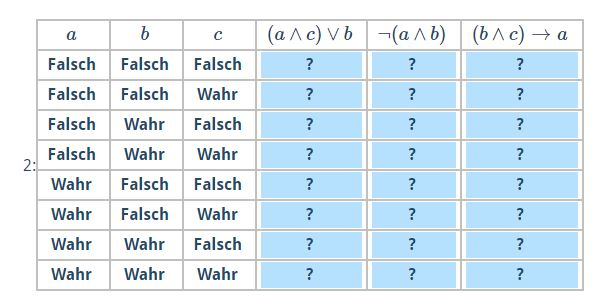Index

Working with MUMIE as author

Working with MUMIE as teacher

Using MUMIE via plugin in local LMS

FAQ for examination lecturers

Overview

Go back to the old wiki

We revise and update this wiki. We apologize for the inconvenience this may cause you.

# TruthTable

With the answer type input.truth-table you can let the user select the truth values of one or multiple logical terms.# Specify the variables used in the terms

First you need to specify the \usedVariables (semicolon separated). The generic framework will generate the values for all variables.

123456...\begin{answer}  \type{input.truth-table}  \usedVars{a;b}\end{answer}... 

# Defining the order of the generated value

As you can see in the picture above the values generated for the variables starts with false for every variable. If you
rather it to start with true instead, you can use the command \top{true}.

1234567...\begin{answer}  \type{input.truth-table}  \usedVars{a;b}  \top{true}\end{answer}... 

# Define the logical terms to be evaluated

Then, you can define the terms (again semicolon separated) that should be displayed as tasks.

1234567...\begin{answer}  \type{input.truth-table}  \usedVars{a;b}  \tasks{$(a \wedge c) \vee b$;$\neg(a \wedge b)$;$(b \wedge c) \rightarrow a$}\end{answer}... 

# Specify the solutions

At last, you need to specify the solutions. Note that the solution has to be hard coded (1 for true, or 0 for false).
The length of the solution depends on the length of usedVars and you need semicolon separated solution for each term.

12345678...\begin{answer}  \type{input.truth-table}  \usedVars{a;b}  \tasks{$(a \wedge c) \vee b$;$\neg(a \wedge b)$;$(b \wedge c) \rightarrow a$}  \solution{00110111;11111100;11101111}\end{answer}... 

# Using variables

Instead of passing the arguments directly, you can also pass in string variables for the \usedVars, \tasks
and \solution commands (see example below):

123456789101112131415...\begin{question}  \begin{variables}    \string{vars}{p;q}    \string{task}{$p \wedge q$;$p \vee q$}    \string{solu}{0001;0111}  \end{variables}  \begin{answer}    \type{input.truth-table}    \usedVars{vars}    \tasks{task}    \solution{solu}  \end{answer}\end{question}... 

# Using variable pool to create variations of the tasks

In order to show random questions and create personalized user data, you can use variables defined in a pool.

12345678910111213141516171819202122232425262728293031\begin{question}  \begin{pool}    \begin{variables}      \string{vars}{p;q}      \string{task}{$p \wedge q$;$p \vee q$}      \string{solu}{0001;0111}    \end{variables}     \begin{variables}      \string{vars}{a;b}      \string{task}{$a \wedge b$;$\neg(a \vee b)$}      \string{solu}{0001;1000}    \end{variables}     \begin{variables}      \string{vars}{s;t}      \string{task}{$\neg t$;$s \vee \neg t$}      \string{solu}{1010;1011}    \end{variables}     ... more ...  \end{pool}  \text{\textbf{fill in the truth table}}  \type{input.generic}   \begin{answer}    \type{input.truth-table}    \usedVars{vars}    \tasks{task}    \solution{solu}  \end{answer} 

More Examples